# 基于Super-Twisting滑模的SRM直接转矩控制Direct Torque Control of Switched Reluctance Motor Based on Super-Twisting Sliding Mode

• 全文下载: PDF(1851KB)    PP.220-226   DOI: 10.12677/CSA.2019.92026
• 下载量: 345  浏览量: 643

Switched reluctance motor (SRM) has many excellent qualities, so how to restrain the torque ripple to make it more widely used and popularized has become a hot issue in academia. Although the traditional direct torque control (DTC) of switched reluctance motor can restrain the torque ripple to some extent, its restraining effect is limited. And also, there are problems such as big overshoot and weak robustness. In this paper, a direct torque controller based on Super-Twisting sliding mode control is designed. In addition, some improvements have been made to the direct torque controller. Finally, the theory was simulated and verified by using MATLAB/Simulink. The results show that, compared with the traditional direct torque control, the direct torque control of the switched reluctance motor based on the sliding mode control effectively improves the control performance of the system, makes the torque ripple smaller and meets the control requirements.

1. 引言

2. Super-twisting滑模控制器设计

$\frac{\text{d}x}{\text{d}t}=a\left(x,t\right)+b\left(x,t\right)u,y=c\left(x,t\right)$ (1)

$u={K}_{A}\mathrm{sgn}{k}_{1}\stackrel{˙}{y}+{k}_{2}{|y|}^{r}\mathrm{sgn}\left(y\right)+{K}_{B}\mathrm{sgn}{k}_{3}\stackrel{˙}{y}+{k}_{4}{|y|}^{r}\mathrm{sgn}\left(y\right)$ (2)

Super-twisting滑模控制是一种二阶滑模控制方案，其控制思想是将开关作用的控制量 $u$ 应用到高阶导数中，这样能有效抑制传统滑模控制中产生的抖振，并能继承传统滑模控制优秀的动态响应和鲁棒性   。本文中将其引入对转速环的控制中。Super-twisting滑模控制律包含了两个部分：一个不连续的滑模变量函数和一个连续导数的函数，分别如式(3)、(4)所示。

$u=-{K}_{p}{|y|}^{r}\mathrm{sgn}\left(y\right)+{u}_{1}$ (3)

$\frac{\text{d}{u}_{1}}{\text{d}t}=-{K}_{I}\mathrm{sgn}\left(y\right)$ (4)

${K}_{p}>\frac{{A}_{M}}{{B}_{m}},{K}_{I}\ge \frac{4{A}_{M}}{{B}_{m}^{2}}\cdot \frac{{B}_{M}\left({K}_{p}+{A}_{M}\right)}{{B}_{m}\left({K}_{p}-{A}_{M}\right)}$ (5)

$\frac{{\text{d}}^{2}y}{\text{d}{t}^{2}}=A\left(x,t\right)+B\left(x,t\right)u$ (6)

${T}_{e}=J\frac{\text{d}\omega }{\text{d}t}+D\omega +{T}_{L}$ (7)

$\frac{{\text{d}}^{2}\omega }{\text{d}{t}^{2}}=-\frac{1}{J}\left(\frac{\text{d}{T}_{L}}{\text{d}t}+D\frac{\text{d}\omega }{\text{d}t}\right)+\frac{1}{J}\frac{\text{d}{T}_{e}}{\text{d}t}$ (8)

${T}_{e}{}^{*}={k}_{p}{|{s}_{\omega }|}^{r}\mathrm{sgn}\left({s}_{\omega }\right)+{T}_{e}$ (9)

$\frac{\text{d}{T}_{e}}{\text{d}t}={k}_{i}\mathrm{sgn}\left({s}_{\omega }\right)$ (10)

3. 改进的直接转矩控制

${U}_{k}={R}_{k}{i}_{k}+\frac{\text{d}{\psi }_{k}}{\text{d}t}$ (11)

$\psi \left(k\right)=\psi \left(k-1\right)+U\left(k\right){T}_{s}$ (12)

$\Delta {\psi }_{s}=|{\psi }_{s}\left(k\right)|-|{\psi }_{s}\left(k-1\right)|\approx |U\left(k\right){T}_{s}|\mathrm{cos}{\theta }_{\upsilon \psi }$ (13)

$\Delta {\psi }_{s-increase}=|V\left(k+1\right)|{T}_{s}\mathrm{cos}{\theta }_{\upsilon \psi 1}{\theta }_{\upsilon \psi 1}\in \left(30˚,90˚\right)$ (14)

$\Delta {\psi }_{s-decrease}=|V\left(k+2\right)|{T}_{s}\mathrm{cos}{\theta }_{\upsilon \psi 2}{\theta }_{\upsilon \psi 2}\in \left(90˚,150˚\right)$ (15)

$\theta \in \left(0˚,20˚\right)$ 时， ${V}_{k+1}$ 对应的夹角 ${\theta }_{\upsilon \psi 1}\in \left(70˚,90˚\right)$${V}_{k+2}$ 对应的夹角 ${\theta }_{\upsilon \psi 2}\in \left(130˚,150˚\right)$ ，根据余弦三角函数的特性可知， $\mathrm{cos}{\theta }_{\upsilon \psi 1}$ 的值比 $\mathrm{cos}{\theta }_{\upsilon \psi 2}$ 的值小得多，这就会导致在一个采样周期内，磁链幅值的增加与减小存在明显的不同，增加量很少但减少量却很多，使得磁链出现明显的不对称性导致出现转矩抖振。同理，当 $\theta \in \left(40˚,60˚\right)$ 时，磁链的增加量明显大于磁链的减小量，而 $\theta \in \left(20˚,40˚\right)$ 时增加量和减小量相差不大。所以根据这种特性，本文将每一扇区以 $20˚$ 为界近一步细分为三个小扇区，则原来的6个传统扇区可重新划分为18个扇区。Table 1. Switching vector table based on 12 sections

4. 系统仿真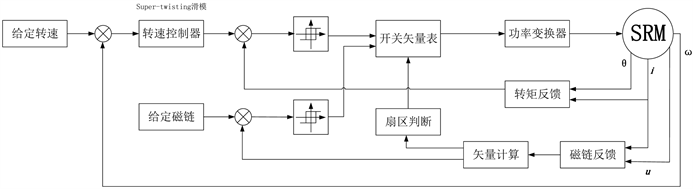Figure 1. Basic principle structure diagram of SRM system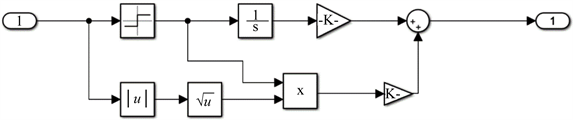Figure 2. Speed control module based on super-twisting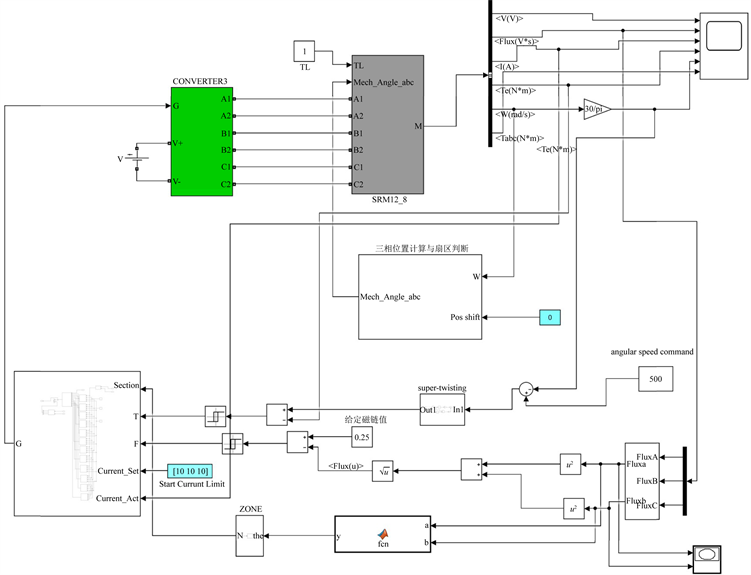Figure 3. Simulation model of SRM direct torque control system based on Super-twisting sliding mode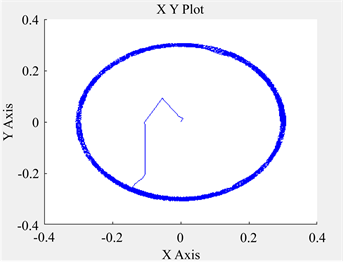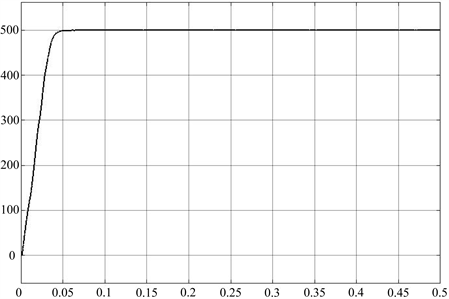Figure 5. Speed waveform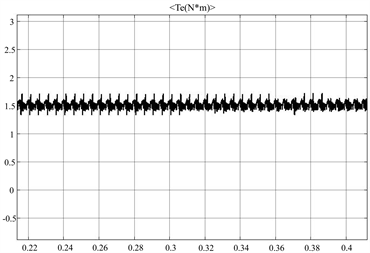(a) 转矩响应波形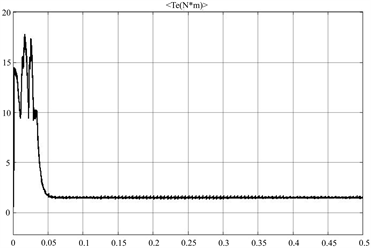(b) 转矩响应波形局部放大

Figure 6. Output torque waveform

5. 结论

  吴红星. 开关磁阻电机系统理论与控制技术[M]. 北京: 中国电力出版社, 2010.  Annegret, K., Andreas, H. and Rik, W. (2017) Direct Instantaneous Torque and Force Control: A Control Approach for Switched Reluctance Machines. IET Electric Power Applications, 11, 935-943. https://doi.org/10.1049/iet-epa.2016.0515  王玉林. 开关磁阻电机直接瞬时转矩控制的研究[D]: [硕士学位论文]. 杭州: 浙江大学, 2016.  王勉华, 邢幸. 开关磁阻电机直接转矩滑模变结构控制[J]. 电机与控制应用, 2017, 44(6): 35-39.  Levant, A. (2007) Principles of 2-Sliding Mode Design. Automatica, 43, 576-586. https://doi.org/10.1016/j.automatica.2006.10.008  Cristian, L. and Ion, B. (2013) Super-Twisting Sliding Mode Control of Torque and Flux in Permanent Magnet Synchronous Machine Drives. 39th Annual Conference of the IEEE Industrial Electronics Society, Vi-enna, 10-13 November 2013, 3171-3176.  Rafiq, M., Rehman, S.U., Rehman, F.U., et al. (2012) A Second Order Sliding Mode Control Design of a Switched Reluctance Motor Using Super Twisting Algorithm. Simulation Modelling Practice & Theory, 25, 106-117. https://doi.org/10.1016/j.simpat.2012.03.001  李鹏, 郑志强. 基于类二次型Lyapunov函数的Super-Twisting算法收敛性分析[J]. 控制与决策, 2011, 26(6): 949-952.